# SETS

A set is a well defined collection of objects.
The collection member is called number, object or element .
We shall denote sets by capital alphabets eg. A,b, ——- &
The elements by the small alphabets e.g- a,b,c,—————- z
Some reserve letters for these sets.
N: for the set of natural numbers {1,2,3,4,5,6,……………….}
Z: for the set of integers {-3,-2,-1,0,1,2,3…………………….}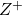: for the set of all positive integers.
Q: for the set of all rational numbers.
T- the set of all irrational number
(x:x =, m,n e z,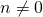) (x:xe r and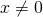): for the set of all positive rational number.
R: for the set of all real numbers.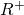: for the set of all positive real numbers.
C: for the set of all complete numbers.

Then NCZCQCR
TCR ANDDescription of sets:- Set is often described in the following two ways.

1) ROSTER METHOD :- a set is described by listing elements,separated by commas, within fraces { } e.g the set of vowels of English alphabets described as (a,e,i,o,u).
2) SET SUICDER METHOD:- in this method a set is described by a characterizing property
P(x) of elements x in such a case the set is described .
{x:P(x) holded} or {x/P(x) holder} is read as
The set of all x such that P(x) holds
e.g:- A= (0,1,2,3,4,8,16,………………..) is written as A = {: xe}

TYPES OF SETS
EMPTY SET:- if it has no elements, said empty or void or nail set & denoted by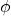in roster methodis denoted by {}
builder method = {xeR := -z}

SINGLE TON SET a set consisting of a single element e.g {5} is a single ton set.
{x:xEN} and=9 } is a single ton set equal to {3}

FINITE SET: A finite set it is either empty set or its element can be listed or counted. By natural number (1,2,3,……….) the process of listing terminates at a certain natural number n(A) and is called ordinal number or order of a finite set

e.g. set A = (1,2,3,………………….99) Natural number less then 100
INFINITE SET : A set whose element cannot be listed by the natural number 1,2,3 …………… n for only natural number n is called an infinite set

Example
set of all paints in a plane.
{ex er : 0 EQUIVALENT SETS: two limit sets A and B are equivalent if their cardinal numbers are same i.e n(A) = n(B)
set A = (1,2,3) set B = {a.b,c,}
Here n(A) = n(B)

EQUAL SETS: if every elements of set A is a number of set Band every elements of set B is a number of set A, are called equal sets

Set A = {1,2,5,6}
Set B = {5,6,2,1}

SUB SETS: if every elements of A is a an element of B, then A is called sub set of B and denoted by“A is sub set of B”
Thusif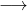The symbole stands for “implies”
If A is not a sub set of B we write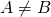SUPER SET : If A is subset of B then B is a super set of A

Note:- 1) every set is a subset of itself2) empty set is subset of every set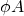3) above 1 and 2 are called improper sub set
4) A subset A of a set B is called a proper sub set of BIf AB then B is super set of A
If A=B then A≤B and5) the total number of subsets of a finite set containing n elements is 2n

A finite set A containing n elements each sub set of A have r elements
Then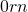element is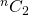then the total number of sub set of A
=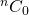+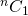+………………………….. +(binomial theorem )

INTERVAL OF SUB SETS OF R

1) CLOSED INTERVELS – on real line a & b two given real numbers such that a is called closed interval & written [a b]E.g.is the set of all real numbers laying between -1 & 2 including -1 and 2 , clearly it is a infinite sub set of R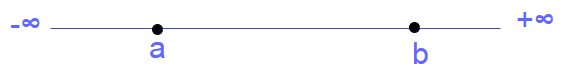2) OPEN INTERVALS – on real line, a & b two given real number such that a < b , then the set of all real number x such that a < x < b is called open interval & is denoted by (a,b) or ]a,b[3) SEMI OPENED OR SEMI CLOSED INTERVAL: on real line, a & b two given real number such that a < x ≤ b or a ≤ x < b is called semi open or semi closed interval & denoted by (a,b] or [a,b) or ]a,b], [a,b[Note:- the number b-a is called the length of any of the intervals. (a,b) [a,b] [a,b) (,b] The interval (0,) denoted by set R+ of all non negative real number
The interval (-,0) denoted by set R- of all negative real number
The interval (-,) denoted by set R of all real number

UNIVERSAL SET:a set that contains all sets under consideration i.e. it is a super set of each of the given set, is called universal set & is denoted by U Thus a set that contains all set in a given contex is called the universal.
e.g A= {1,2,3}, B = {2,4,5,6}, c= {1,3,5,7,}
then u = {1,2,3,4,5,6,7}

e.g. when we are using intervals on real line, the set R of real numbers is taken as the universal set.

POWER SET: the collection or family of all sub sets of a is called the power set & is denoted by p(A) = {s:s CA}

Let set A = {1,2,3}
Then the sub set are-, {1}, {2}, {3},{1,2}, {1,3}, {2,3}, {1,2,3}
= P(A) (a set having n elements has 2n sub set s)
(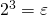sub sets)

Venn Diagram tutorials